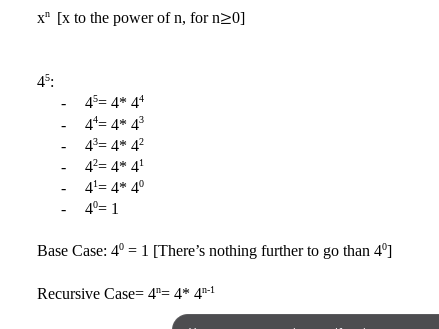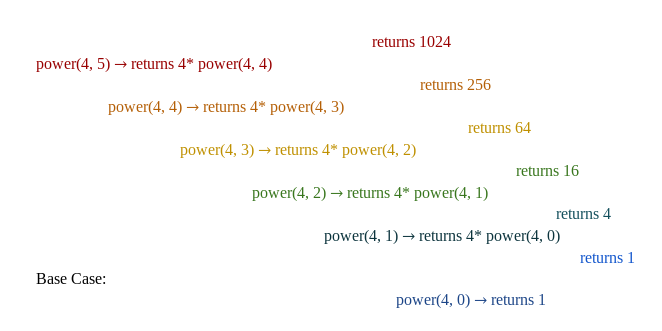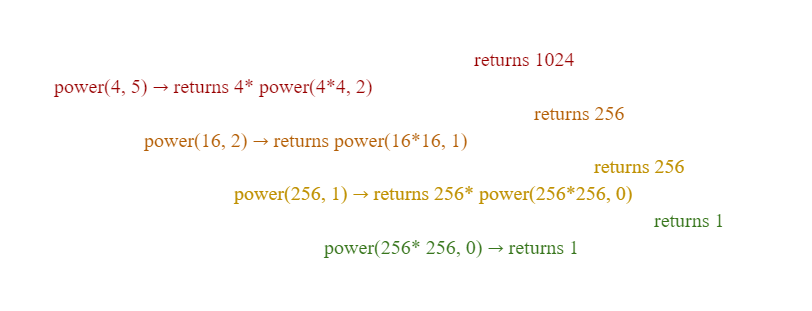×

Search anything:

# Recursion in Java

#### Java recursionGet this book -> Problems on Array: For Interviews and Competitive Programming

#### This article will dive into the basic introduction to recursion, and how it is implemented in Java.

1. What is Recursion?
2. Rules to Recursion
3. Recursion in Content
4. Pros and Cons of Recursion
5. Conclusion

## What is Recursion?

Recursion is the use of the word inside the definition, or in other words, the function calls itself.

While this may seem confusing at a glance, recursion is actually a very convenient way to organize and simplify your code to make it easier for you and others to understand. Like a for loop, recursion also follows certain conditions to end the function loop, as to avoid an infinite loop, and contrary, it can perform at a better running time analysis than a for loop.

## Rules to Recursion

To avoid an infinite loop, a recursive function needs to follow certain criterion:

1. a base case

Base Case- the basic output of the function that should not call the function, but rather return what is defined by the function (int, String, float, etc.) because there's nothing more to call recursively

Recursive Case- calls the function itself until it reaches the base case. The function called should have a value in the parameter that will slowly lead to the base case.

## Recursion in Content

To help better understand recursion and how it is implemented, we will use basic math equations in the real world, and solve it recursively.

For example, let's say we want to find x^n, where n is a real number greater than or equal to 0:
The base case will always be x to the zero power, because any number to the zero power is one. Therefore, for the first part of the recursion, we can write the code with a condition statement checking if n is equal to zero, and return 1.

Since we learned that exponents is just x multiplied by itself n times, same as multiplication, where x* n is x plus itself n times, the recursive case can be as follows:
x^n= x* x^(n-1)
x^(n-1)= x* x^(n-2)
...
All the way until it reached the base case.

We can see this in the example shown below.In the above image, 4^5 is used as an example

Translating it to code, we could write it as:

``````public class Recursion{
public static void main(String[] args){

System.out.println(power(4,5));
}

public static int power( int x, int n){
//Base Case
if (n==0){
return 1;}
//Recursive Case
return x* power(x, n-1);
}
}
``````

Step by step implementation of code:In the above image, we can see the steps to what each of the code returns

Including the negative exponents, the code can be revised as:

``````public class Recursion{
public static void main(String[] args){

System.out.println(power(4,-5));
}

public static double power( double x, int n){
//Base Case
if (n==0){
return 1;}

if (n> 0){
return x* power(x, n-1);
}
else{
return 1/x *  power(x, n+1);
}
}
}
``````

Time for a pop quiz:
What will be the recursive case for the factorial of x?

• 0!
• 1!
• x* (x-1)!
• x!

Ans: x* (x-1)!
Example: 4!= 4* 3!
3!= 3* 2!
2!= 2* 1!
1!= 1* 0!
0!= 1 //Base Case

## Pros and Cons of Recursion

### Pros

• Recursion simplifies the code by calling itself, therefore minimizes the time needed to debug the code if an error occurred.

• If implemented correctly, recursion can reduce the time complexity needed to run the code compared to iteration

• In the recursion code for power above, the code runs linearly by a function of θ(n). By comparing this recursion to a for loop, we notice that there is no significant change in the running time function.
``````public class Recursion{
public static void main(String[] args){

int x= 4;
int n= 5;
int exp= 1;
for(int i= 0; i< n; i++){
exp*=x;
}
System.out.println(exp);
}
}
``````

Running time function of For loop runs linearly θ(n)

However, we can fix this by improving the code of the recursive function.

``````public class Recursion{
public static void main(String[] args){

System.out.println(power(4,-5));
}
public static int power(int x, int n){
if (n==0){
return 1;}
else if (n%2 ==0){
return power(x*x, n/2);
}
else
return x* power(x*x, n/2);
}
}
``````

How this works:Again, we use 4^5 as an example.
To understand this, we need to look at the addition rules for exponents, in which 4^5 can be simplified as 4^2* 4^2* 4, hence we divide n by two. Therefore, the first recursion calls returns 4* power(4^2, 2).
By looking at the running time analysis of this function, we could see, from the picture above, that the steps to the result improved significantly as compared to the first time, and since the function is called recursively with n divided by 2, the function can be said to have a running time θ(log_2(n)).

### Cons

• Recursion requires more memory as every time the function calls itself it adds on to the stack.
• It is a bit confusing to understand mentally because the function basically calls itself, and unless you write the code out step by step, you will get lost in the process of understanding how the code works in more complex situations.
• It is a bit more difficult to write, as opposed to for loops, because you will have to find a base case and a recursive case that leads to the base case. Not all functions are recursive, and a function is only recursive if the terms follows the same pattern to the base case.

## Conclusion

In this article at OpenGenus, we have looked at how Recursion is implemented to solve simple problems, like x to the power of n, as the definition of this function is recursive, x^n= x* x^(n-1), leading to the base case. We have also seen the pros and cons of recursion and how to write recursive code effectively as to minimize our running time analysis.

However, recursion can do beyond more than the simple scope of mathematics. In a more complex scenario, like a Binary Tree, recursion is used to list the tree in order of preorder, postorder, and inorder.